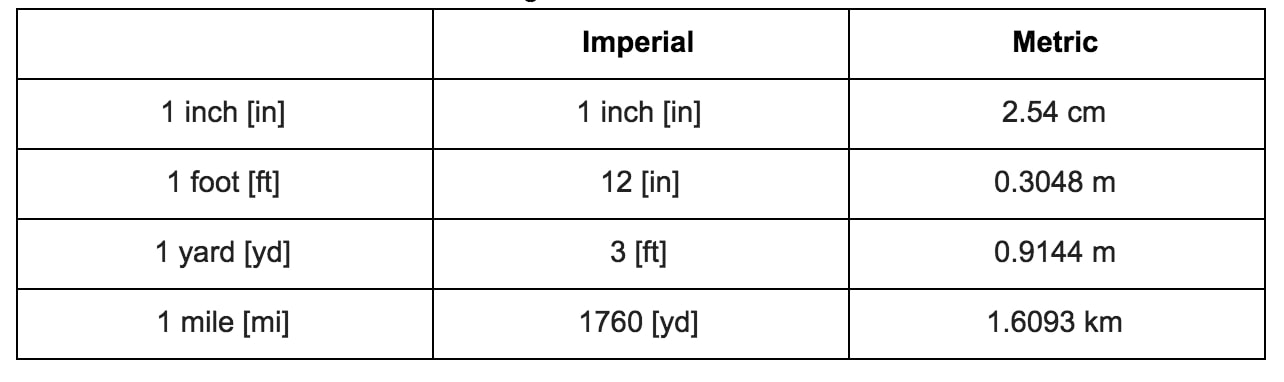# Imperial systems

## Imperial conversions

The imperial system is used in Britain and some other Commonwealth countries. That's why it's sometimes also known as the British imperial system. The imperial system is not based on the number of 10, unlike the metric system. In this lesson we're going to learn how to convert between different imperial units.

## Foot to inches

What is 1 foot? How many inches in a foot is there? A foot is equalled to 12 inches on the imperial system. Each inch is equalled to 2.54 centimeters if you're more familiar with the metric system. If you're converting feet to inches, remember to multiply the number of inches by 12. If you're converting inches to feet, you'll have to divide by 12 to get to the corresponding number of feet.

## Yard to feet

How many foot in a yard is there? 1 yard is equalled to 3 feet. Again, if you're more familiar with the metric system, each yard is equalled to 0.9144 meters. When you're converting yard to feet, multiply the number of yards by 3 to get the total feet. On the other hand, you'll have to divide by 3 in order to convert feet to yards.

## Mile to yards

How many yards in a mile is there? 1 mile is the equivalent of 1760 yards. In metric terms, that's 1.6093 kilometers. For each mile that you're converting into yards, you'll have to multiply by 1760. If you're trying to change yards into miles, then divide it by 1760 to get the equivalent miles.

Here's a summary imperial conversion table of the above information for your reference.

Length Conversion RatesChart of length conversion rates

## Example questions

Question 1

Converting the imperial units

1a) 43 ft =_____ yd

Since we know that 1 yard equals 3 feet, we'll have to divide 43 by 3 in order to find the equivalent yards.

$43 ft \bullet \frac{1 yd}{3 ft} = 14.33yd$

1b) 530 in =____ mi

When you've got inches and you want to turn it into miles, work back through the different unit conversions in order to find your answer. First, we can convert 530 inches into feet. Therefore, we'll have to divide 530 by 12. We'll then convert the feet to yards. This means we'll have to divide the number we get by 3 in order to get yards. Lastly, convert the yards that we get to miles. To do this, we'll have to divide by 1760 yards.

In other words, we can write the following:

$530 in \bullet \frac{1 ft}{12 in} \bullet \frac{1 yd}{3 ft} \bullet \frac{1 mi}{1760 yd} = 14.72 mi$

1c) 50 yd =____ mi

Checking back to the reference chart, we know that 1760 yards equals 1 mile. Therefore, we'll have to divide 50 by 1760 in order to convert our 50 yards into miles.

$50 yd \bullet \frac{1 mi}{1760 yd} = 0.028 mi$

Question 2

Add or subtract different imperial units

2a) 250 in + 345 yd - 0.005 mi =____ ft

Convert each unit into feet.

$250 in \bullet \frac{1 ft}{12 in} = 20.3 ft$

$345 yd \bullet \frac{3 ft}{1 yd} = 1035 ft$

$0.004 mi \bullet \frac{1760 yd}{1 mi} \bullet \frac{3 ft}{1 yd} = 26.4 ft$

Plug back into original equation and solve for the length in feet.

$20.3 ft + 1035 ft - 26.4 ft = 1029.43 ft$

2b) 0.03 mi + 400 ft - 5000 in =____ yd

$0.03 mi \bullet \frac{1760 yd}{1 mi} = 52.8 yd$

$400 ft \bullet \frac{1 yd}{3 ft} = 133.3 yd$

$5000 in \bullet \frac{1 ft}{12 in} \bullet \frac{1 yd}{3 ft} = 138.8 yd$

Plug back into original equation and solve for the length in yard.

$52.8 yd + 133.3 yd - 138.8 yd = 47.2 yd$

2c) 0.3 mi - 500 ft + 2345 in =____ mi =____ yd =____ ft

$0.3 mi \bullet \frac{1760 yd}{1 mi} = 528 yd$

$500 ft \bullet \frac{1 yd}{3 ft} = 166.7 yd$

$2345 in \bullet \frac{1 ft}{12 in} \bullet \frac{1 yd}{3 ft} = 65.14 yd$

Plug it back into the original equation and solve for answer in yards

$528 yd - 166.7 yd + 65.14 yd = 426 yd$

Convert answer to miles and feet.

$426 yd \bullet \frac{1 mi}{1760 yd} = 0.242 mi$

$426 yd \bullet \frac{3 ft}{1 yd} = 1270 ft$

If you wanted to double check your answers, check out this online unit converter dealing with lengths. Here's also another general unit converter you may find handy.

If you were stuck at any point during this lesson, you may want to go back and review several lessons that build up to this imperial conversion class. Review how to multiply fractions and whole numbers, proper fractions, improper fractions, and decimals. Ratios and rates are also lessons that touches on converting numbers.

### Imperial systems

Imperial system, also known as the "British Imperial", is mostly used in Britain and some other Commonwealth countries. Unlike metric units, imperial units are not based on the number of 10. Some of the most commonly used imperial units are inch, pound and yard.

#### Lessons

$\cdot$ Mainly used in US and some in Canada
$\cdot$ Not based on number of 10

Lengths
1 foot (ft) = 12 inches (in)
1 yard (yd) = 3 feet (ft)
1 mile (mi) = 1760 yards (yd)
• Introduction
a)
What are Imperial systems?

• 1.
Converting the imperial units
a)
43 ft = _____ yd

b)
530 in = _____ mi

c)
50 yd = _____ mi

• 2.
Add or subtract different imperial units
a)
250 in + 345 yd - 0.005 mi = _____ ft

b)
0.03 mi + 400 ft - 5000 in = _____ yd

c)
0.3 mi - 500 ft + 2345 in = ____ mi = _____ yd = ______ ft